American Journal of Applied Mathematics
Volume 4, Issue 4, August 2016, Pages: 175-180

New Predictor-Corrector Iterative Methods with
Twelfth-Order Convergence for Solving Nonlinear Equations

Noori Yasir Abdul-Hassan

Department of Mathematics, College of Education for Pure Sciences, University of Basrah, Basrah, Iraq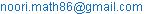Noori Yasir Abdul-Hassan. New Predictor-Corrector Iterative Methods with Twelfth-Order Convergence for Solving Nonlinear Equations. American Journal of Applied Mathematics. Vol. 4, No. 4, 2016, pp. 175-180. doi: 10.11648/j.ajam.20160404.12

Received: May 16, 2016; Accepted: May 31, 2016; Published: June 17, 2016

Abstract: In this paper, we propose and analyze new two efficient iterative methods for finding the simple roots of nonlinear equations. These methods based on a Jarratt's method, Householder's method and Chun&Kim's method by using a predictor-corrector technique. The error equations are given theoretically to show that the proposed methods have twelfth-order convergence. Several numerical examples are given to illustrate the efficiency and robustness of the proposed methods. Comparison with other well-known iterative methods is made.

Keywords: Nonlinear Equations, Predictor-Corrector Methods, Convergence Analysis, Efficiency Index, Numerical Examples

Contents

1. Introduction

The problem of solving a single nonlinear equation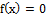is fundamental in various branches of science and engineering. Recently, there are many numerical iterative methods have been developed to solve these problems. These methods are constructed by using several different techniques, such as Taylor series, quadrature formulas, homotopy perturbation technique and its variant forms, decomposition technique, variational iteration technique, and Predictor-corrector technique. For more details, see [1-4,6,32]. In this paper, we use the Predictor-corrector technique to construct some new iterative methods based on a Jarratt's method as a predictor with Householder's method and Chun &Kim's method as a correctors. The orders of convergence and corresponding error equations of the obtained iteration formulae are derived analytically to show that our proposed methods have twelfth -order convergences. Each one of these methods requires two evaluations of the function, three evaluations of first-derivative and one evaluations of second-derivative per iteration. Therefore, our proposed methods have the same efficiency index is 121/6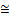1.51309. To illustrate the performance of these new methods, we give several examples and a comparison with other well-known iterative methods is given.

2. Preliminaries

Definition 2.1 (see [12, 32]): Let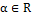,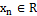, n = 0, 1, 2,…. Then the sequence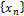is said to converge to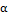if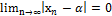. If, in addition, there exist a constant c≥ 0, an integer n0 ≥ 0 and p ≥ 0 such that for all n>n0,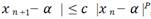, thenis said to be convergence to α with convergence order at least p. If p = 2 or 3, the convergence is said to be quadratic or cubic respectively.

Notation 2.1: Let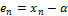is the error in the nth iteration. Then the relation(2.1)

is called the error equation for the method. By substitutingfor all n in any iterative method and simplifying, we obtain the error equation for that method. The value of p obtained is called order of convergence of this method which produces the sequence.

Definition 2.2 (see [3,6]): Efficiency index is simply defined as

E.I.=p1/m (2.2)

Where p is the order of the method and m is the number of functions evaluations required by the method (units of work periteration).

3. Construction of the Method

In this section, we recall some of the important methods such as Newton’s method, Jarratt’s method, Halley's method, Householder’s method Chun & Kim's method and Jarratt-Halley's method in the following six Algorithms:

Algorithm (3.1): For a given x0, compute approximates solution xn+1 by the iterative scheme: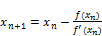(3.1)

This is the well-known Newton's method, which has a quadratic convergence . Its efficiency is 1.41421.

Algorithm (3.2): For a given x0, compute approximates solution xn+1 by the iterative scheme: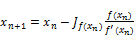(3.2)

Where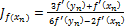and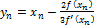. This is known as Jarratt’s fourth-order method [4, 8]. Its efficiency is 1.58740.

Algorithm (3.3): For a given x0, compute approximates solution xn+1 by the iterative scheme: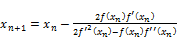(3.3)

This is known as Halley's method [9,10,11,13,23], which has cubic convergence and its efficiency is 1.44225.

Algorithm (3.4): For a given x0, compute approximates solution xn+1 by the iterative scheme: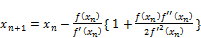(3.4)

This is known as Householder’s method, which has cubic convergence . Its efficiency is 1.44225.

Algorithm (3.5): For a given x0, compute approximates solution xn+1 by the iterative scheme: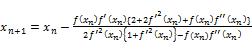(3.5)

This is the third order method was referred by Chun and Kim [6,14]. Its efficiency is 1.44225.

Algorithm (3.6): For a given x0, compute approximates solution xn+1 by the iterative scheme: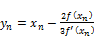(3.6.a)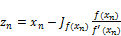(3.6.b)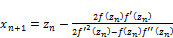(3.6.c)

Where. This is known as Jarratt-Haley's method , which has twelfth-order of convergence. Its efficiency is 1.513086.

Now, we present the following new two predictor-corrector iterative methods which have twelfth-order convergence, based on a combination scheme between Jarratt's method and each one of Householder's method and Chun&Kim's method, by using Algorithm (3.2) as a predictor and Algorithm (3.4) and Algorithm (3.5) as a corrector, for solving the nonlinear equation f(x)=0.

Algorithm (3.7): For a given x0, compute approximates solution xn+1 by the iterative scheme:(3.7.a)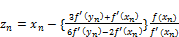(3.7.b)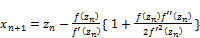(3.7.c)

This is called the predictor-corrector Jarratt-Householder's method (JHHM), which has twelfth-order of convergence. Its efficiency is 1.513086.

Algorithm (3.8): For a given x0, compute approximates solution xn+1 by the iterative scheme:(3.8.a)(3.8.b)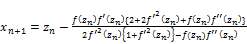(3.8.c)

This is called the predictor-corrector Jarratt-Chun&Kim's method (JCKM), which has twelfth-order of convergence. Its efficiency is 1.513086.

4. Convergence Analysis of the Methods

In this section, we compute the orders of convergence and corresponding error equations of the proposed methods (Algorithm (3.7) and Algorithm (3.8)) as follow.

Theorem 4.1: Let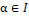be a simple zero of sufficiently differentiable function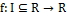for an open interval I. If x0 is sufficiently close to, then the iterative method defined by Algorithm (3.7) is of order twelve and it satisfies the following error equation:

en+1= (c34c23- 5c33c25+ 9c32c27- (1/729)c3c43- 7c3c29+ (2/729)c43c22+ (2/27)c42c25+(2/3)c4c28+ 2c211- (1/9)c3c42c23-(5/3)c3c4c26

+ (1/27)c42c2c32- (1/3)c4c33c22+ (4/3)c4c32c24)e12+ O(e13)  (4.1)

Where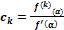, k=2, 3, …, and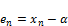(4.2)

Proof: Let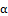be a simple zero of f. Then by expanding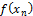and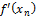in Taylor’s series about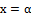, we get

f(xn) =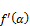{e+ c2e2 + c3e3+ c4e4+ c5e5+…}  (4.3)={1+ 2c2e+ 3c3e2+ 4c4e3+ 5c5e4+6c6e5+…}  (4.4)

From (4.3) and (4.4), we have= e- c2e2+ (-2c3+2c22)e3+ (7c2c3-3c4-4c23)e4+ (10c2c4-4c5+6c32-20c3c22+8c24)e5    (4.5)

Also,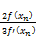= (2/3)e- (2/3)c2e2+ (-(4/3)c3+ (4/3)c22)e3+ ((14/3)c2c3-2c4- (8/3)c23)e4+ ((20/3)c2c4- (8/3)c5

+ 4c32- (40/3)c3c22+ (16/3)c24)e5+…  (4.6)

Substituting (4.6) and (4.2) into (3.7.a), and simplifying, we have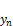=α+(1/3)e+ (2/3)c2e2+ ((4/3)c3- (4/3)c22)e3+ (-(14/3)c2c3+ 2c4+ (8/3)c23)e4+ (-(20/3)c2c4

+ (8/3)c5-4c32+ (40/3)c3c22- (16/3)c24)e5+…  (4.7)

From (4.7), using Taylor's expansion and simplifying, we have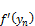={1+ (2/3)c2e+ ((4/3)c22+ (1/3)c3)e2+ (4c2c3+ (4/27)c4 -(8/3)c23)e3+ ((44/9)c2c4- 32/3)c3c22

+ (8/3)c32+ (16/3)c24)e4+ ((52/9)c4c3- (40/3)c4c22+ (16/3)c2c5- 12c2c32+ (80/3)c3c23- (32/3)c25)e5 +… }  (4.8)

Combining (4.8) and (4.4), using Taylor's expansion and simplifying, we have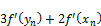= 4+ 4c2e+ (4c22+4c3)e2+ ((40/9)c4+ 12c2c38c23)e3 + (5c5+ (44/3)c2c4-32c3c228c32+ 16c24)e4

+ ((52/3)c4c3- 40c4c22+ 16c2c5- 36c2c32+ 80c3c23- 32c25+ 6c6)e5+ …  (4.9)

Also,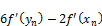= 4+ (8c22-4c3)e2+ (-(64/9)c4+ 24c2c3- 16c23)e3+ (-10c5+(88/3)c2c4- 64c3c22+ 16c32+ 32c24)e4

+ ((104/3)c4c3- 80c4c22+ 32c2c5- 72c2c32+ 160c3c23-64c25-12c6)e5+ …  (4.10)

Dividing (4.9) by (4.10), using Taylor's expansion and simplifying, we have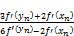= 1+ c2e+ (-c22+2c3)e2+ ( (26/9)c4- 2c2c3)e3+ (- (17/9)c2c4- 3c3c22+ 2c24+ (15/4)c5)e4

+ (-(3/2)c2c5- (44/9)c4c22+14c3c23- 9c2c32- 4c25+ (19/9)c4c3+ (9/2)c6)e5+… (4.11)

Combining (4.5) and (4.11), using Taylor's expansion and simplifying, we have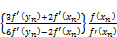= e+ (c2c3-c23- (1/9) c4) e4+ ((20/9) c2c4- (1/4)c5+ 2c32- 8c3c22+ 4c24)e5 +…  (4.12)

Substituting (4.12) and (4.2) into (3.7.b), and simplifying, we have

zn= α+ (-c2c3+c23+ (1/9) c4) e4+ (-(20/9) c2c4+ (1/4) c5-2c32+8c3c22-4c24) e5+ …   (4.13)

From (4.13), using Taylor's expansion and simplifying, we have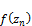={(-c2c3+ c23+ (1/9) c4) e4+ (-(20/9)c2c4+ (1/4) c5-2c32+ 8c3c22- 4c24)e5+ …}  (4.14)

And,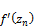={1+ (-2c22c3+2c24+ (2/9) c2c4) e4- ((40/9) c22c4-(1/2) c2c5+ 4c2c32- 288c3c23+ 144c25) e5+ …}  (4.15)

Also,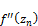={2c2+ (-6c2c32+6c3c23+ (2/3) c3c4) e4- ((40/3) c2c3 c4-(3/2) c3c5+12c33-48c32c22+ 24c3c24) e5+ …}   (4.16)

From (4.14), (4.15) and (4.16), using Taylor's expansion and simplifying, we have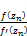= (-c2c3+ c23+(1/9) c4) e4+ (-(20/9) c2c4+ (1/4) c5- 2c32+ 8c3c22- 4c24) e5+ …  (4.17)

And,

1+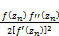= 1+ (-c22c3+c24+(1/9) c2c4) e4- ((20/9) c22c4- (1/4) c2c5+ 2c2c32- 8c3c23+ 4c25) e5+ …  (4.18)

Combining (4.17) and (4.18), using Taylor's expansion and simplifying, we have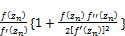= (-c2c3+c23+ (1/9) c4) e4+ (-(20/9) c2c4+ (1/4) c5- 2c32+ 8c3c22- 4c24) e5+ …   (4.19)

Thus, substituting (4.13) and (4.19) into (3.7.c), using Taylor's expansion and simplifying, we have

xn+1 =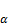+ (c34c23- 5c33c25+ 9c32c27- (1/729)c3c43- 7c3c29+ (2/729)c43c22+ (2/27)c42c25+ (2/3)c4c28 +2c211

-(1/9)c3c42c23- (5/3)c3c4c26+ (1/27)c42c2c32- (1/3)c4c33c22+ (4/3)c4c32c24)e12+ O(e13) (4.20)

Which implies that

en+1 = (c34c23- 5c33c25+ 9c32c27- (1/729)c3c43- 7c3c29+ (2/729)c43c22+ (2/27)c42c25+ (2/3)c4c28+ 2c211

- (1/9)c3c42c23- (5/3)c3c4c26+ (1/27)c42c2c32- (1/3)c4c33c22+ (4/3)c4c32c24)e12+ O(e13) (4.21)

This is show that Algorithm (3.7) is twelve-order convergent.

Theorem 4.2: Let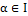be a simple zero of sufficiently differentiable functionfor an open interval I. If x0 is sufficiently close to, then the iterative method defined by Algorithm (3.8) is of order twelve and it satisfies the following error equation:

en+1= (c34c23- (9/2)c33c25+ (15/2)c32c27- (1/729)c3c43- (11/2)c3c29+ (1/486)c43c22+ (1/18)c42c25+ (1/2)c4c28

+ (3/2)c211+ (1/27)c42c2c32- (1/3)c4c33c22+ (7/6)c4c32c24-(5/54)c3c42c23- (4/3)c3c4c26)e12+ O(e13)   (4.22)

Proof. Similar procedure, to the proof of theorem 4.1, can be applied to analyze the convergence of Algorithm (3.8).

5. Numerical Examples

In this section, we present the results of numerical calculations on different functions and initial points to demonstrate the efficiency of proposed methods, Jarratt- Householder's method (JHHM) and Jarratt- Chun&Kim's method (JCKM). Also, we compare these methods with the classical Newton’s method (NM) and other methods, as Jarratt's method (JM), Halley's method (HM), Householder's method (HHM), Chun&Kim's method (CKM), and Jarratt-Halley's method (JHM). All computations are carried out with double arithmetic precision. We use the stopping criteria |xn+1 xn| < ϵ and |f (xn+1)|< ϵ, where ϵ = 1015, for computer programs. All programs are written in MATLAB.

Different test functions and their approximate zeros x* found up to the 15th decimal place are given in Table 1, the efficiency index (E.I.) of various iterative methods is given in Table 2 and the number of iterations (NITER) to find x* is given in Table 3. NC in Table 3 means that the method does not converge to the root x*.

Table 1. Different test functions and their approximate zeros (x*).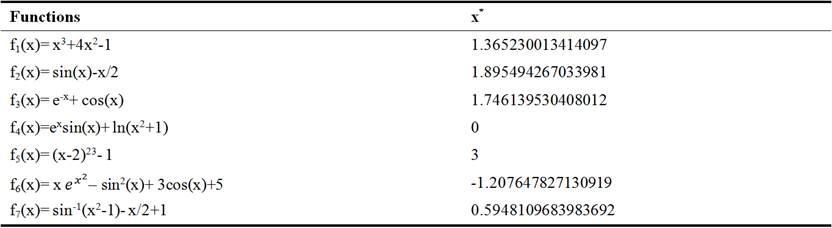Table 2. Comparisons between the methods depending on the efficiency index (E.I.).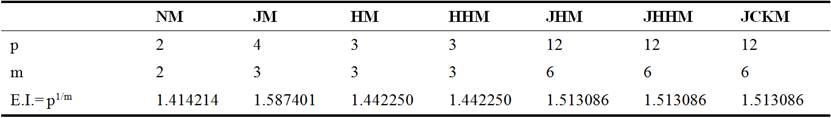Table 3. Comparisons between the methods depending on the number of iterations (NITER).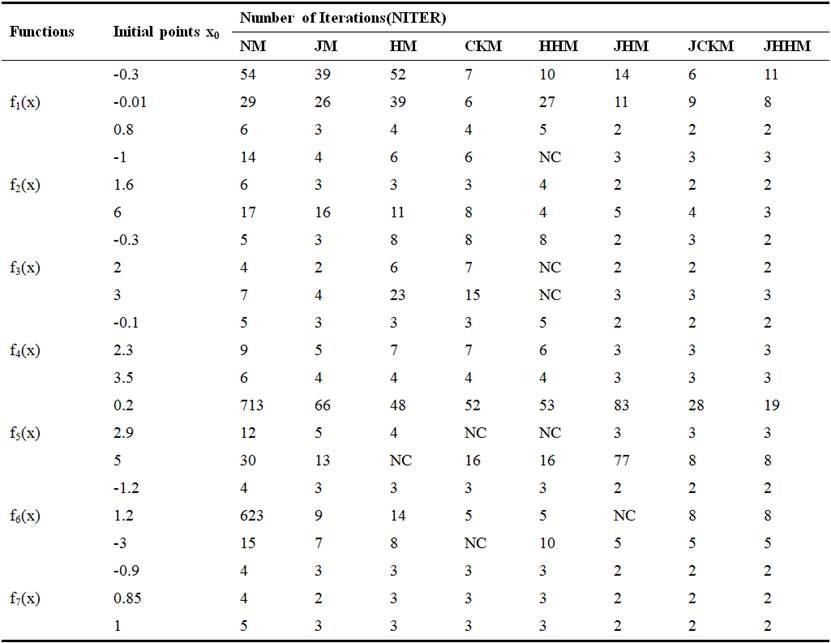6. Conclusion

In this paper, we presented new two predictor-corrector iterative methods with twelfth-order convergence for solving nonlinear equations, which are based on the Jarratt's method, Householder's method and Chun & Kim's method. The proposed methods have the same efficiency index is equal to 1.513086. From numerical experiments we show that our methods are efficient, robust and faster convergence in comparison with classical Newton's method and some other methods.

References

1. Abbasbandy, S., Improving Newton–Raphson Method for Nonlinear Equations by Modified Adomian Decomposition Method, Appl. Math. Comput. 145, (2003): 887-893.
2. Ahmad F., S. Hussain, S. Hussain, A. Rafiq, New Twelfth-Order J-Halley Method for Solving Nonlinear Equations, Open Science Journal of Mathematics and Application, 1(1), 2013: 1-4.
3. Amat, S., Busquier, S., Gutiérrez, J. M., Geometric Construction of Iterative Functions to Solve  Nonlinear Equations, J. Comput. Appl. Math. 157, (2003): 197-205.
4. Argyros, I. K., Chen, D., Qian, Q., The Jarratt Method in Banach Space Setting, J. Comput. Appl. Math., 51, (1994): 1-3.
5. Burden, R. L. and Faires, J. D., Numerical Analysis, 9th edition, Brooks/Cole PublishingCompany, 2011.
6. Chun, C. and Kim, K., Several New Third-Order Iterative Methods for Solving NonlinearEquations, Acta Application Mathematicae, 109(3), (2010): 1053-1063.
7. Chun, C., Iterative Methods Improving Newton’s Method by the Decomposition Method, Comput. Math., Appl. 50, (2005): 1559-1568.
8. Chun, C., Some Improvements of Jarratt’s Methods with Sixth-Order Convergences, Appl. Math. Comput. 190, (2007): 1432-1437.
9. Ezquerro, J. A., Hernandez, M. A., A Uniparametric Halley-Type Iterationwith Free Second Derivative, Int. J. pure Appl. Math. 6 (1), (2003): 103-114.
10. Ezquerro, J. A., Hernandez, M. A., On Halley-type iterations with Free Second Derivative, J. Comput. Appl. Math. 170, (2004): 455-459.
11. Gutiérrez, J. M., Hernández, M. A., An Acceleration of Newton's Method:Super-Halley Method,  Appl. Math. Comput. 117 (2001): 223-239.
12. Hadi, T., New on Spline Functions for SolvingNonlinear Equations, Bullettin of Mathematical Analysis and Applications, 3(4), (2011): 31-37.
13. Halley, E., A New Exact and Easy Method of Finding the Roots of Equations Generally and that  without any Previous Reduction, Philos. Trans. R. Soc. London, 18, (1694):136–148.
14. Ham, Y. M., Chun, C. and Lee, S. G., Some Higher-Order Modifications of Newton’s Method for Solving Nonlinear Equations, J. Comput. Appl. Math. 222, (2008): 477-486.
15. Hasan A., Srivastava, R. B., Ahmad, N., An Improved Iterative Method Based on Cubic Spline Functions for Solving Nonlinear Equations, 4(1),(2014): 528-537.
16. Jarratt, P., Some Fourth Order Multipoint Iterative Methods for SolvingEquations, Math. Comput., 20 (95), (1966): 434-437.
17. Jayakumar, J. and Kalyanasundaram M., Power Means Based Modification of Newton’s Method for Solving Nonlinear Equations with Cubic Convergence, Int. J. Appl. Math. Comput. 6(2), (2015): 1-6.
18. Khattri, S. K., Quadrature Based Optimal Iterative Methods with Applicationsin High-Precision Computing, Numer. Math. Theor. Meth. Appl., 5, (2012):592-601.
19. Kou, J., and Li, Y., The Improvements of Chebyshev-Halley Methods with Fifth-Order Convergence, Appl. Math. Comput. 188 (1), (2007): 143-147.
20. Kou, J., and Li, Y., An Improvement of The Jarratt Method, Appl. Math. Comput. 189(2), (2007): 1816-1821.
21. Kumar, S., Kanwar, V., and Singh, S., Modified Efficient Families of Two and Three-Step Predictor- Corrector Iterative Methods for Solving Nonlinear Equations, Applied Mathematics, 1, (2010): 153-158.
22. Li, Y. T. and Jiao, A. Q., Some Variants of Newton’s Method with Fifth-Order and Fourth-Order Convergence for Solving Nonlinear Equations, Int. J. Appl. Math. Comput., 1, (2009): 1-16.
23. Melman, A., Geometry and Convergence of Halley’s Method, SIAM Rev. 39 (4), (1997): 728-735.
24. Noor, K. I. and Noor, M. A., Predictor-Corrector Halley Method for Nonlinear Equations, Appl. Math. Comput., 188 (2007): 1587-1591.
25. Noor, K. I., Noor, M. A. and Momani, S., Modified Householder Iterative Method for Nonlinear Equations, Appl. Math. Comput. 190 (2007): 1534-1539.
26. Noor, M. A. and Khan, W. A., New Iterative Methods for Solving Nonlinear Equation by Using Homotopy Perturbation Method, Appl. Math. Comput. 219(2012): 3565-3574.
27. Noor, M. A., Khan, W. A. and Younus, S., Homotopy PerturbationTechnique for Solving Certain Nonlinear Equations, Appl. Math. Sci., 6(130), (2012): 6487-6499.
28. Noor, M. A., Iterative Methods for Nonlinear Equations Using Homotopy Perturbation Technique, Appl. Math. Inform. Sci. 4(2), (2010): 227-235.
29. Noor, M. A., Some Iterative Methods for Solving Nonlinear Equations Using Homotopy Perturbation Method, Int. J. Comp. Math., 87, (2010): 141-149.
30. Oghovese, O., John, E., Some New Iterative Methods Based on Composite Trapezoidal Rule for Solving Nonlinear Equations, IJMSI, 2(8), (2014): 1-6.
31. Saeed, K. R. and Aziz, M. K., Iterative Methods for Solving Nonlinear Equations by Using Quadratic Spline functions, Mathematical Sciences Letters, 2(1), (2013): 37-43.
32. Weerakoon, S., Fernando, T. G. I., A Variant of Newton’s Method with Accelerated Third- Order Convergence, Applied Mathematics Letters, 13(8), (2000): 87-90.

 Contents 1. 2. 3. 4. 5. 6.
Article ToolsAbstractPDF(235K)